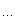# Brian Warehouse

Some birds aren`t meant to be caged, their feathers are just too bright... ...
posts - 40, comments - 16, trackbacks - 0, articles - 1
f(n) 是正整数n 的各位数字之和。如果 f(n) 是1位数那么n的数根就是f(n)，否则f(n) 的数根就是n 的数根。举例说明：987的数根是6 (9+8+7=24 2+4=6)。你的任务是找出这样表达式的数根: A1*A2*…*AN + A1*A2*…*AN-1 + … + A1*A2 + A1。

(A+B)mod C = (A mod C) + (B mod C)
(AB) mod C = (A mod C) × (B mod C)

C编译器 , 0MS  0KB , 如果你把前两句定义变量的语句对调，将会耗费 20MS 的时间
#include <stdio.h>
int main()
{

int i=0,j,K,N,temp,m;

long int A;
scanf(
"%d",&K);

for (; i<K; i++) {
scanf(
"%d",&N);
m
=1;
A
=0;

for (j=0; j<N; j++) {
scanf(
"%d",&temp);
temp
%=9;
m
=(m*temp)%9;
A
=(A+m)%9;
}
printf(
"%d\n",(A+8)%9+1);
}

return 0;
}

posted @ 2010-08-19 15:35 Brian 阅读(252) | 评论 (0)编辑 收藏

#include <iostream>
#include
<stdio.h>
using namespace std;

int main()
{
__int64 x,left,right,mid;
scanf(
"%I64d",&x);
left
=0;
right
=x+1;

while ((left+1)<right) // 二分查找
{
mid
=(left+right)/2;

if(mid*mid<=x)
left
=mid;

else
right
=mid;
}
printf(
"%I64d",left);

return 0;
}

posted @ 2010-08-19 14:36 Brian 阅读(650) | 评论 (3)编辑 收藏posted @ 2010-08-19 00:23 Brian 阅读(215) | 评论 (0)编辑 收藏

C++ 编译器 ，220K  0MS
#include<iostream>
#include
<climits> // 为第一组数据运算做的约束
using namespace std;
int RGB,in,out; // in 用来接收数据, out 用来暂存映射正确的数据

int main(){

for (int i=0; i<16; i++// 输入的数据中前16组是 target set
cin>>RGB[i]>>RGB[i]>>RGB[i];

while (1) {
cin
>>in>>in>>in; // 开始接收映射组
if (in[0== -1break;

int MIN=INT_MAX; // 2147483647
for (int i=0; i<16; i++) {

int sum=(RGB[i]-in)*(RGB[i]-in)+
(RGB[i][
1]-in)*(RGB[i]-in)+
(RGB[i][
2]-in)*(RGB[i]-in); // 不需要开方，开方易产生误差
if (sum < MIN) {

out=RGB[i];

out=RGB[i];

out=RGB[i];
MIN
=sum; // 最小的即映射成功
}
}
cout
<<"("<<in<<","<<in<<","<<in<<") maps to ("

<<out<<","<<out<<","<<out<<")"<<endl;
}

return 0;
}

posted @ 2010-08-18 01:00 Brian 阅读(388) | 评论 (0)编辑 收藏

DescriptionInput

Output

V2=iR=CR d/dt (VS*cos(wt)-VR*cos(wt+q))=VRcos(wt+q)
= CR w (sin(wt+q)-sin(wt))=VRcos(wt+q)// C 编译器:#include <stdio.h>#include
<math.h>int main(){int i=0,n;double VR,VS,R,C,w;scanf(
"%lf%lf%lf%d",&VS,&R,&C,&n);for (; i<n; i++){scanf(
"%lf",&w);VR
=C*R*w*VS / sqrt(1+C*C*R*R*w*w);printf(
"%.3lf\n",VR);}return 0;}

posted @ 2010-08-17 14:49 Brian 阅读(899) | 评论 (0)编辑 收藏

#include <iostream>
#include
<string>
using namespace std;

int main()
{

int a,b,c={0},i,j,ls1,ls2;

string s;

for (cin>>s,ls1=s.length(),i=ls1-1,j=0; i>=0; i--)
a[j
++]=s[i]-'0'//将第一个数逆序放入a数组

for (cin>>s,ls2=s.length(),i=ls2-1,j=0; i>=0; i--)
b[j
++]=s[i]-'0'//将第二个数逆序放入b数组

for (i=0; i<ls1; i++)

for (j=0; j<ls2; j++)
{
c[i
+j] += a[i]*b[j];

if(c[i+j] >= 10)
{
c[i
+j+1+= c[i+j]/10;
c[i
+j] %= 10;
}
}

i
=399;

while (i--)

if (c[i]) break//跳过所有前导0
for (; i>=0; i--)
printf(
"%d",c[i]); //输出主体部分
return 0;
}

posted @ 2010-08-17 14:24 Brian 阅读(392) | 评论 (0)编辑 收藏

#include <iostream>
#include
<string>
using namespace std;
int main()
{

int a={0},b={0},i,j,len,ls1,ls2,f=0// 相加后结果放在a内
string s;

for (cin>>s,ls1=s.length(),i=ls1-1,j=0; i>=0; i--)
a[j
++]=s[i]-'0'//将第一个数逆序放入a数组

for (cin>>s,ls2=s.length(),i=ls2-1,j=0; i>=0; i--)
b[j
++]=s[i]-'0'//将第二个数逆序放入b数组

for (i=0,len=ls1>ls2?ls1:ls2; i<len; i++// 注意len取二者较大的值
{
a[i]
+= b[i]; //相加结果放入a数组
if (a[i] >= 10)
{
a[i]
%= 10//进位处理
a[i+1]++;
}
}

if (a[len]) printf("%d",a[len]); //所谓的前导0
for (i=len-1; i>=0; i--)
printf(
"%d",a[i]); //输出主体部分
return 0;
}

posted @ 2010-08-17 14:21 Brian 阅读(281) | 评论 (0)编辑 收藏

```500 3
150 300
100 200
470 471
```

`298`
`基本思路：将所有的树做标记，移走则标记为0，存在标记为1.由于不好判断给定数目是多少，故用向量。效率虽然不高，但是可以AC。`
```#include <iostream>#include <vector>using namespace std;int main(){    int L,M,i=0,j,start,end,count=0;    scanf("%d%d",&L,&M);    vector<int> Mark(L+1,1);        for (; i<M; i++)     {        scanf("%d%d",&start,&end);        for (j=start; j<=end; j++)            Mark[j]=0;    }    for (i=0; i<=L; i++)        if (Mark[i]==1)            count++;    printf("%d\n",count);    return 0;}
```
` `

posted @ 2010-08-17 14:19 Brian 阅读(581) | 评论 (0)编辑 收藏

Description

Input

Output

n行，每行输出对应一个输入。

Sample Input

```2
100000
111
```

Sample Output

```20
7
```
`09小孩问我的一道题，原来写的代码足足有90多行，今天重写：`
```
#include <iostream>#include <string>using namespace std;int main(){    int n,pos,sec,i,j,w = {1,2,4,8}; //sec是分段处理,pos是对应权值位置    char x = "0123456789ABCDEF"; //打表            string bin; //输入的二进制字符串    cin>>n;    while (n--)    {        cin>>bin;        sec=bin.length()%4;        pos=0;        for (i=sec; i>0; i--)            if (bin[sec-i]=='1')                pos += w[i-1];        if (sec) printf("%c",x[pos]);        for (i=sec; i<bin.length(); i+=4)        {            pos=0;            for (j=0; j<4; j++)                if (bin[i+j]=='1')                    pos += w[3-j];            printf("%c",x[pos]);        }        printf("\n");    }    return 0;}
```

posted @ 2010-08-17 14:18 Brian 阅读(275) | 评论 (0)编辑 收藏

Description

Input

Output

Sample Input

```2 12345
3 67890
0 0
```

Sample Output

```      --   --        --
|    |    | |  | |
|    |    | |  | |
--   --   --   --
| |       |    |    |
| |       |    |    |
--   --        --
---   ---   ---   ---   ---
|         | |   | |   | |   |
|         | |   | |   | |   |
|         | |   | |   | |   |
---         ---   ---
|   |     | |   |     | |   |
|   |     | |   |     | |   |
|   |     | |   |     | |   |
---         ---   ---   ---
```

Hint

#include <iostream>
using namespace std;

char n1[] = "- -- -----";
char n2[] = "|   ||| ||";
char n3[] = "|||||  |||";
char n4[] = "  ----- --";
char n5[] = "| |   | | ";
char n6[] = "|| |||||||";
char n7[] = "- -- -- --";

int main()
{

int m;

char n;
cin
>>m>>n;

while ((n-'0')||m)
{

int len = strlen(n);

for (int i=0;i<len;i++)
{
cout
<<" ";

int num = n[i]-'0';

for (int j=0;j<m;j++)
{
cout
<<n1[num];
}
cout
<<"  ";
}
cout
<<endl;

int temp = m;

while (temp--)
{

for (int i=0;i<len;i++)
{

int num = n[i]-'0';
cout
<<n2[num];

for (int j=0;j<m;j++)
{
cout
<<" ";
}
cout
<<n3[num];
cout
<<" ";
}
cout
<<endl;
}

for (int i=0;i<len;i++)
{
cout
<<" ";

int num = n[i]-'0';

for (int j=0;j<m;j++)
{
cout
<<n4[num];
}
cout
<<"  ";
}
cout
<<endl;
temp
= m;

while (temp--)
{

for (int i=0;i<len;i++)
{

int num = n[i]-'0';
cout
<<n5[num];

for (int j=0;j<m;j++)
{
cout
<<" ";
}
cout
<<n6[num];
cout
<<" ";
}
cout
<<endl;
}

for (int i=0;i<len;i++)
{
cout
<<" ";

int num = n[i]-'0';

for (int j=0;j<m;j++)
{
cout
<<n7[num];
}
cout
<<"  ";
}
cout
<<endl<<endl;

cin
>>m>>n;
}

return 0;
}

posted @ 2010-08-17 14:17 Brian 阅读(409) | 评论 (0)编辑 收藏